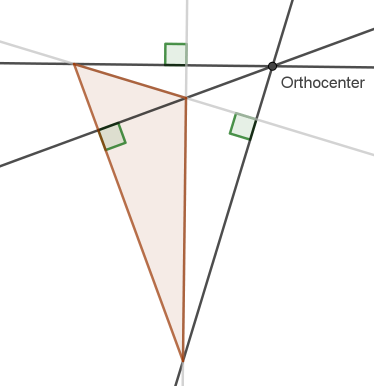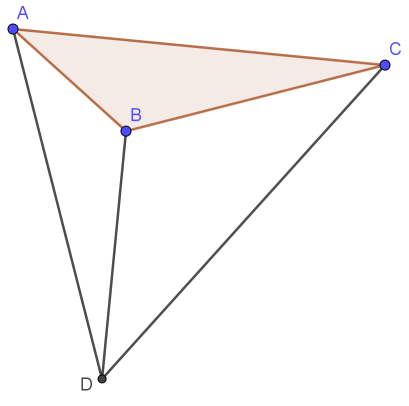# Math question about the orthocenter of a triangle

In my geometry class, when I get to the section on triangle “centers”, there are three main ones that have good mathematical and practical utility that make for wonderful application: the circumcenter (center of a circle containing all three vertices), incenter (center of largest inscribed circle), and the centroid (actual balance point of any triangle). But when I get to a fourth center - the orthocenter (which is the concurrent intersection of all three extended altitude lines of any triangle) I have thus far been stymied to offer up any use, application, or even mathematical utility for this particular center. [And yes - I know about the Euler Line which includes the orthocenter in its interesting set - but I only know that as a wonderful mathematical factoid to have fun with more than an application toward anything further.]

I usually take the occasion to tease students with the ongoing challenge mathematicians aspire to, to find something so totally cool and yet so challengingly useless that nobody is able to apply or use it toward anything else - and how mathematicians have been foiled at pretty much every turn because somebody will end up brilliantly using their hopeful conjecture or new theorem as a springboard toward something practical or some further mathematical utility.

So I also have to include the obligatory humility that I’m pretty sure the orthocenter probably does have a use (multiple ones I shouldn’t wonder) and that I’m just as-of-yet ignorant of them. Not that I’ve spent any large portion of my hours officially researching this - but simple internet searches haven’t turned up anything satisfactory for me. And some quick results just yield up answers from people who are mistaken about what they’re talking about (pretty sure the person answering this question was confusing ‘orthocenter’ with ‘circumcenter’ - the more proper answer to the question posed. …though the glider answer given further down might be intriguing).

As you can see, the orthocenter is one of those ‘centers’ that doesn’t even have to remain in the interior of the actual triangle, as seen in the obtuse triangle example shown below. Does anybody out there happen to know of some architectural or engineering significance - or even further mathematical utility that the orthocenter of a triangle manages to fulfill? I offer that up here as a fun amusement to ponder.Well, it would be a good name for an orthopedic hospital…

3 Likes

Thanks for this. I never got to teach geometry but it was always my favorite area of math owing to how visually oriented I am. Words are so clumsy by comparison. Look forward to playing around with this.

So the measures of center in question involve the intersection of the perpendiculars from the midpoints or the angle bisectors, or, the center of the circle containing the vertices or the center of the circle intersecting each side in exactly one point. And the first one is the one of interest here since it is in need of an application. This will have to percolate a while since we’re having our big Zoom Christmas party this afternoon, also known as the big Hannukah party by our Jewish friends.

Genuinely lol’d out loud.

1 Like

Yeah … so many centers (and ways to find them). My students are (in theory) busy cramming this stuff for their upcoming final. Which is the first obvious real-world application of the orthocenter. It is fair game to include on Mr. B’s geometry final. For some reason, though, my students don’t consider that as a legitimate answer to the “where is this ever used” question!

Summary:
Circumcenter: intersection of perpendicular bisectors of each side
Incenter: intersection of angle bisectors of each interior angle
Centroid: intersection of medians (segments connecting each vertex to midpoint of opposite side)
Orthocenter: intersection of altitudes (extended as may be necessary to said point of intersection)

Okay! So if three arbitrary cities on a map all want to find the best place for their shared orthopedic hospital, we now know just where it should go! Brilliant, Phil!2 Likes

From here I learned a curious fact that maybe useful in your exploration. It seems that when you make a triangle with the orthocenter and two of the vertices of the triangle, then the orthocenter of the new triangle is the vertex you left out. The result is that ether the orthocenter divides a triangle with all acute angles into three triangles each with one obtuse angles OR you find the triangle with all acute angles which your triangle with one obtuse angle is one of the parts which that triangle is divided into by its orthocenter.

This means every triangle either has all acute angles or is a part of a triangle with all acute angles using the orthocenter. It sounds to me like this could be useful in the developments of proofs about triangles.

Also, I have done a lot of numerical analysis and one method uses triangles to search a surface for things and I have to wonder if this cannot be used in such an algorithm.

2 Likes

That’s a good observation. I’ve noticed those sorts of patterns myself too. It ends up making what looks like a top view of a pyramid. Point D below is the orthocenter of ABC, but then point B is also the orthocenter of ACD and so forth.So the orthocenter of Dallas, Austin, and Houston would be near the small town of Noak Texas (fairly close to Austin).

Which would mean those three cities must nearly form a right triangle with each other, because only on right triangles does the orthocenter actually coincide with a vertex (the right angle vertex). …depending on how close Noak is to Austin, of course.

If I were still in the algebra trade you can bet I’d want them to appreciate this approach.

It is just the quadratic formula rewritten as d +/- sqrt(d^2 - c) where d = -b/(2a)

here is another example x^2 - 9x + 18 = 0

a = 1, b = -9, c = 18

So d= 4.5 and you have 4.5 +/- sqrt(20.25 - 18) = 4.5 +/- sqrt(2.25) = 4.5 +/- 1.5 = 3 and 6

then you want the product (4.5 - p)(4.5 + p) = 18 or 20.25 - p^2 = 18 or p^2 = 2.25. so p = 1.5
which means your 2 numbers are 4.5 - p = 3 and 4.5 + p = 6.

For some students that rational will make it easier and for others will seem unnecessarily convoluted. People are different and learn in different ways, and a 1 size fits all approach is going to make some students struggle.

I found this, excerpted below, some of it interesting:

That’s interesting. I immediately made up an example that was pretty similar to yours, x^2 - 9x - 10 = 0

What I like about it is the process is pretty intuitive throughout and there is no need to derive the quadratic equation at all, though I think it is a cool thing in its own right. But as a method to employ it isn’t all that great. Many students get that even when simple factoring doesn’t get it done, completing the square is often less bother. The problem with that is some students don’t have the ready number sense to make completing the square an attractive option. This new (to me) way of solving quadratics seems to require less facility with finding two numbers which relate in two ways while remaining intuitive in a way that plugging numbers in the quadratic formula is not.

The need for the quadratic equation is a compact way of writing down the same method which people like me find much easier to remember. One way of putting it is to ask whether the student has better visual or better audio / language memory. For me the former is much better than the latter. The quadratic equation is visually compact and much easier for me to remember than a load of audio / language instructions.

Wrong is cutting a sentence in half and then suggesting as a fix what was stated in the other half, albeit less formally. You sure are obsessed being right and finding others wrong.

2 Likes

This topic was automatically closed 6 days after the last reply. New replies are no longer allowed.

“Let your conversation be always full of grace, seasoned with salt, so that you may know how to answer everyone.” -Colossians 4:6

This is a place for gracious dialogue about science and faith. Please read our FAQ/Guidelines before posting.